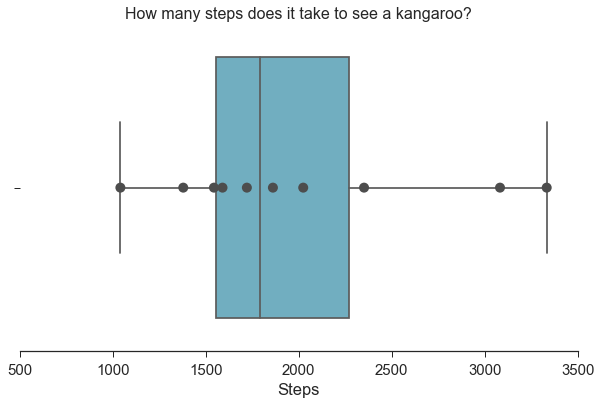How many steps does it take to see a kangaroo? I started wondering this soon after moving to the center of the Australian Outback. So, with the assistance of my Fitbit, I started recording the number of steps I took after walking out the front door until I saw a kangaroo.

Steps
0 3082
1 2024
2 3333
3 1544
4 1861
5 1590
6 1041
7 2351
8 1721
9 1379

It turns that out it took an average of 1992 steps, with a standard deviation of 734. The minimum was 1041 steps and the maximum was 3333.

# Now let's plot it using seaborn
# Make it large
fig, ax = plt.subplots(figsize=(10,6))

# Set some style preferences
sns.set(style="ticks", palette="deep", color_codes=True, font_scale=1.5)

# Create the boxplot
ax = sns.boxplot(x="Steps", data=df,
whis=np.inf, color="c")

# Add in points to show each observation
sns.stripplot(x="Steps", data=df,
size=10, color=".3", linewidth=0)
ax.set_xticks(ax.get_xticks())
sns.despine(trim=True)
plt.title('How many steps does it take to see a kangaroo?', size = 16)

Text(0.5,1,'How many steps does it take to see a kangaroo?')